## The Butterfly, the Gyroid and the Neutrino

#### August 13, 2015

Some butterflies have shiny, vividly colored wings. From different angles you see different colors. This effect is called iridescence. How does it work?

It turns out these butterfly wings are made of very fancy materials! Light bounces around inside these materials in a tricky way. Sunlight of different colors winds up reflecting off these materials in different directions.

We're starting to understand the materials and make similar substances in the lab. They're called photonic crystals. They have amazing properties.

Here at the Centre for Quantum Technologies we have people studying exotic materials of many kinds. Next door, there's a lab completely devoted to studying graphene: crystal sheets of carbon in which electrons can move as if they were massless particles! Graphene has a lot of potential for building new technologies — that's why Singapore is pumping money into researching it.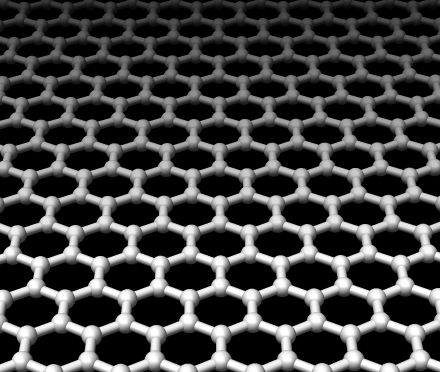Some physicists at MIT just showed that one of the materials in butterfly wings might act like a 3d form of graphene. In graphene, electrons can only move easily in 2 directions. In this new material, electrons could move in all 3 directions, acting as if they had no mass.

The pictures here show the microscopic structure of two materials found in butterfly wings: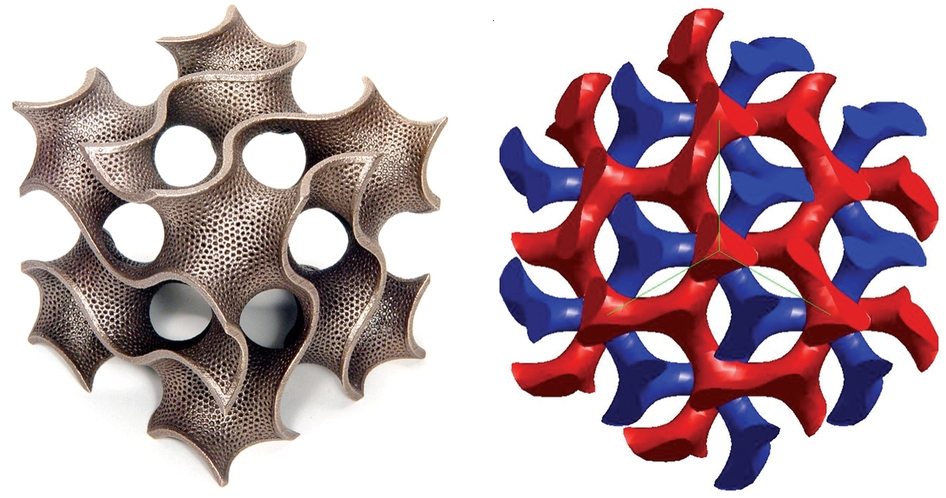The picture at left actually shows a sculpture made by the mathematical artist Bathsheba Grossman. But it's a piece of a gyroid: a surface with a very complicated shape, which repeats forever in 3 directions. It's called a minimal surface because you can't shrink its area by tweaking it just a little. It divides space into two regions.

The gyroid was discovered in 1970 by a mathematician, Alan Schoen. It's a triply periodic minimal surfaces, meaning one that repeats itself in 3 different directions in space, like a crystal.Schoen was working for NASA, and his idea was to use the gyroid for building ultra-light, super-strong structures. But that didn't happen. Research doesn't move in predictable directions.

In 1983, people discovered that in some mixtures of oil and water, the oil naturally forms a gyroid. The sheets of oil try to minimize their area, so it's not surprising that they form a minimal surface. Something else makes this surface be a gyroid — I'm not sure what.

Butterfly wings are made of a hard material called chitin. Around 2008, people discovered that the chitin in some iridescent butterfly wings is made in a gyroid pattern! The spacing in this pattern is very small, about one wavelength of visible light. This makes light move through this material in a complicated way, which depends on the light's color and the direction it's moving.

So: butterflies have naturally evolved a photonic crystal based on a gyroid!

The universe is awesome, but it's not magic. A mathematical pattern is beautiful if it's a simple solution to at least one simple problem. This is why beautiful patterns naturally bring themselves into existence: they're the simplest ways for certain things to happen. Darwinian evolution helps out: it scans through trillions of possibilities and finds solutions to problems. So, we should expect life to be packed with mathematically beautiful patterns... and it is.

The picture at right above shows a 'double gyroid'. Here it is again: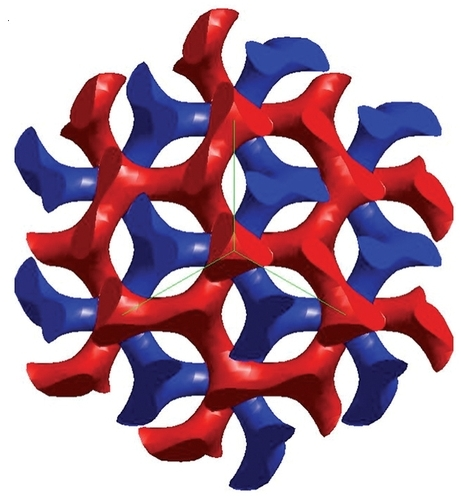This is actually two interlocking surfaces, shown in red and blue. You can get them by writing the gyroid as a level surface:

$$f(x,y,z) = 0$$ and taking the two nearby surfaces $$f(x,y,z) = \pm c$$ for some small value of $$c$$.

It turns out that while they're still growing, some butterflies have a double gyroid pattern in their wings. This turns into a single gyroid when they grow up!

The new research at MIT studied how an electron would move through a double gyroid pattern. They calculated its dispersion relation: how the speed of the electron would depend on its energy and the direction it's moving.

An ordinary particle moves faster if it has more energy. But a massless particle, like a photon, moves at the same speed no matter what energy it has. The MIT team showed that an electron in a double gyroid pattern moves at a speed that doesn't depend much on its energy. So, in some ways this electron acts like a massless particle.

But it's quite different than a photon. It's actually more like a neutrino! You see, unlike photons, electrons and neutrinos are spin-1/2 particles. Neutrinos are almost massless. A massless spin-1/2 particle can have a built-in handedness, spinning in only one direction around its axis of motion. Such a particle is called a Weyl spinor. The MIT team showed that a electron moving through a double gyroid acts approximately like a Weyl spinor!

How does this work? Well, the key fact is that the double gyroid has a built-in handedness, or chirality. It comes in a left-handed and right-handed form. You can see the handedness quite clearly in Grossman's sculpture of the ordinary gyroid: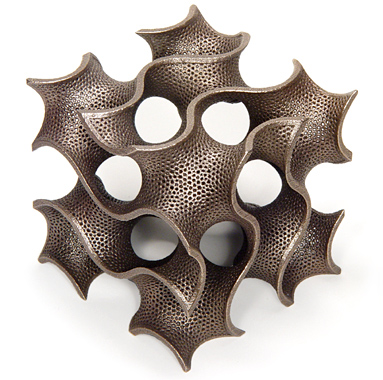Beware: nobody has actually made electrons act like Weyl spinors in the lab yet. The MIT team just found a way that should work. Someday someone will actually make it happen, probably in less than a decade. And later, someone will do amazing things with this ability. I don't know what. Maybe the butterflies know!

### References and more

For a good introduction to the physics of gyroids, see:

For some of the history and math of gyroids, see Alan Schoen's webpage:

For more on gyroids in butterfly wings, see:

The paper by Michielsen and Stavenga is free online! They say the famous blue Morpho butterfly shown in the picture at the top of this page does not use a gyroid. Instead, it uses a "two-dimensional photonic crystal slab consisting of arrays of rectangles formed by lamellae and microribs." But they find gyroids in four other species: Callophrys rubi, Cyanophrys remus, Pardes sesostris and Teinopalpus imperialis. It compares tunnelling electron microscope pictures of slices of their iridescent patches with computer-generated slices of gyroids. The comparison looks pretty good to me: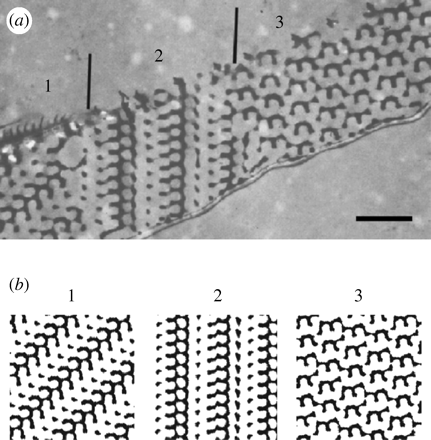For the evolution of iridescence, see:

For the new research at MIT, see:

Again, the first is free online. There's a lot of great math lurking inside, most of which is too mind-blowing too explain quickly. Let me just paraphrase the start of the paper, so at least experts can get the idea:

Two-dimensional (2d) electrons and photons at the energies and frequencies of Dirac points exhibit extraordinary features. As the best example, almost all the remarkable properties of graphene are tied to the massless Dirac fermions at its Fermi level. Topologically, Dirac cones are not only the critical points for 2d phase transitions but also the unique surface manifestation of a topologically gapped 3d bulk. In a similar way, it is expected that if a material could be found that exhibits a 3d linear dispersion relation, it would also display a wide range of interesting physics phenomena. The associated 3D linear point degeneracies are called 'Weyl points'. In the past year, there have been a few studies of Weyl fermions in electronics. The associated Fermi-arc surface states, quantum Hall effect, novel transport properties and a realization of the Adler–Bell–Jackiw anomaly are also expected. However, no observation of Weyl points has been reported. Here, we present a theoretical discovery and detailed numerical investigation of frequency-isolated Weyl points in perturbed double-gyroid photonic crystals along with their complete phase diagrams and their topologically protected surface states.

Also a bit for the mathematicians:

Weyl points are topologically stable objects in the 3d Brillouin zone: they act as monopoles of Berry flux in momentum space, and hence are intimately related to the topological invariant known as the Chern number. The Chern number can be defined for a single bulk band or a set of bands, where the Chern numbers of the individual bands are summed, on any closed 2d surface in the 3d Brillouin zone. The difference of the Chern numbers defined on two surfaces, of all bands below the Weyl point frequencies, equals the sum of the chiralities of the Weyl points enclosed in between the two surfaces.

This is a mix of topology and physics jargon that may be hard for pure mathematicians to understand, so let me try to explain a bit.

For starters, a 'monopole of Berry flux in momentum space' is a poetic way of talking about a twisted complex line bundle over the space of allowed energy-momenta of the electron in the double gyroid. We get a twist at every 'Weyl point', meaning a point where the dispersion relations look locally like those of a Weyl spinor when its energy-momentum is near zero. Near such a point, the dispersion relations are a Fourier-transformed version of the Weyl equation.

The Weyl equation describes a massless left-handed spin-1/2 particle in 3+1-dimensional spacetime, meaning one whose spin points in the same direction as its momentum. This equation is: $$\sigma^\mu \partial_\mu \psi = 0$$ where $$\sigma^\mu$$ are Pauli matrices and $$\psi : \mathbb{R}^4 \to \mathbb{C}^2$$ is a 2-component spinor function on 3+1-dimensonal Minkowski spacetime. Taking the Fourier transform we get $$\sigma^\mu p_\mu \widehat{\psi}(p) = 0$$ where $$p$$ is the energy-momentum 4-vector. Some standard fiddling shows that $$\widehat{\psi}$$ needs to live on the lightcone in energy-momentum space: $$\{ p \in \mathbb{R}^4 : \; p_0^2 - p_1^2 - p_2^2 - p_3^2 = 0 \}$$ which the above paper seems to be calling the 'Dirac cone'.

So, we've got a $$\mathbb{C}^2$$ valued function $$\widehat{\psi}$$ on this cone... but the equation $$\sigma^\mu p_\mu \widehat{\psi}(p) = 0$$ restricts the values this function can take at each point $$p$$, forcing it to live in a 1-dimensional subspace... except at the origin $$p = 0$$, which is called a 'Weyl point'.

So, we have a 1-dimensional complex vector bundle on the lightcone minus this Weyl point, and the cool part is that this bundle is nontrivial! It has a 'twist' at the Weyl point.

The forwards-pointing part of this lightcone with its tip removed is topologically a 2-sphere times a line, and the line is contractible, so we're really getting a nontrivial complex line bundle on the 2-sphere. There's one such bundle for each integer: the 'Chern number', which counts the twistiness of the bundle. The bundle we're getting is the one numbered either +1 or -1, depending on whether our spin-1/2 particle is right-handed or left-handed!

(I wrote down the right-handed version of the Weyl equation, but there's also a left-handed version, where we do something like take the complex conjugates of the Pauli matrices. This particles whose spin points in the opposite direction from their axis of motion.)

If you look in different directions your eyes gaze along different rays in the lightcone, and the possible ways of gazing form a sphere, the 'heavenly sphere', which can be seen as the Riemann sphere. You can also see points in this sphere as different possible directions that the energy-momentum of a massless particle can point. The Weyl equation picks out nontrivial complex line bundle on this sphere!

One thing this implies is that as we continuously change the parameters describing a condensed matter system, Weyl points can't appear or disappear on their: they can only appear or disappear in cancelling pairs. So if I have a condensed matter system that has some sort of left-handed massless spin-1/2 quasiparticle, and I start heating it up or something, that can't make this type of particle no longer possible. It might, however, make a new type of left-handed quasiparticle possible, along with a corresponding new type of right-handed quasiparticle.